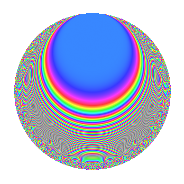# Properties

 Label 231.2.pLevel 231 Weight 2 Character orbit p Rep. character $$\chi_{231}(10,\cdot)$$ Character field $$\Q(\zeta_{6})$$ Dimension 32 Newform subspaces 1 Sturm bound 64 Trace bound 0

# Related objects

## Defining parameters

 Level: $$N$$ = $$231 = 3 \cdot 7 \cdot 11$$ Weight: $$k$$ = $$2$$ Character orbit: $$[\chi]$$ = 231.p (of order $$6$$ and degree $$2$$) Character conductor: $$\operatorname{cond}(\chi)$$ = $$77$$ Character field: $$\Q(\zeta_{6})$$ Newform subspaces: $$1$$ Sturm bound: $$64$$ Trace bound: $$0$$

## Dimensions

The following table gives the dimensions of various subspaces of $$M_{2}(231, [\chi])$$.

Total New Old
Modular forms 72 32 40
Cusp forms 56 32 24
Eisenstein series 16 0 16

## Trace form

 $$32q + 12q^{4} - 12q^{5} + 16q^{9} + O(q^{10})$$ $$32q + 12q^{4} - 12q^{5} + 16q^{9} + 2q^{11} - 32q^{14} + 8q^{15} - 20q^{16} + 8q^{22} + 24q^{23} - 24q^{26} - 12q^{31} - 18q^{33} + 24q^{36} - 32q^{37} + 24q^{38} - 24q^{42} - 28q^{44} - 12q^{45} + 24q^{47} - 36q^{49} + 36q^{53} - 56q^{56} + 12q^{58} - 48q^{59} + 8q^{64} - 36q^{66} + 20q^{67} + 24q^{70} + 72q^{71} + 24q^{75} - 48q^{78} + 72q^{80} - 16q^{81} - 48q^{82} + 64q^{86} + 24q^{88} + 60q^{89} - 28q^{91} - 16q^{92} + 16q^{93} + 4q^{99} + O(q^{100})$$

## Decomposition of $$S_{2}^{\mathrm{new}}(231, [\chi])$$ into newform subspaces

Label Dim. $$A$$ Field CM Traces $q$-expansion
$$a_2$$ $$a_3$$ $$a_5$$ $$a_7$$
231.2.p.a $$32$$ $$1.845$$ None $$0$$ $$0$$ $$-12$$ $$0$$

## Decomposition of $$S_{2}^{\mathrm{old}}(231, [\chi])$$ into lower level spaces

$$S_{2}^{\mathrm{old}}(231, [\chi]) \cong$$ $$S_{2}^{\mathrm{new}}(77, [\chi])$$$$^{\oplus 2}$$

## Hecke Characteristic Polynomials

There are no characteristic polynomials of Hecke operators in the database